## How to Calculate and Solve for the Perimeter or Circumference, Diameter, Radius and Angle of a Sector | The Calculator Encyclopedia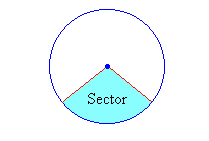The image above is a sector.

To compute the Perimeter or Circumference a sector, two essential parameters is needed and this parameters are the radius of the sector (r) and the angle of the sector (θ). You can also use the diameter of the sector (d).

The formula for calculating the Perimeter or Circumference of a sector:

P = 2r + θ / 360(2πr)

Where;

P = Perimeter or Circumference
r = Radius of the sector
θ = Angle of the Sector

Let’s solve an example:
Find the perimeter or circumference of a sector when the radius of the sector is 14 cm and the angle of the sector is 60°

This implies that;

r = Radius of the sector = 14 cm
θ = Angle of the sector = 60°

P = 2r + θ / 360(2πr)
P = 2 x 14 + 60 / 360(2 x 3.142 x 14)
P = 28 + 0.1667 (87.97)
P = 28 + 14.66
P = 42.66

Therefore, the perimeter or circumference of the sector is 42.66 cm.

Calculating the Perimeter or Circumference of a Sector using Diameter and Angle of the sector.

P = d + θ / 360(πd)

θ = Angle of the sector
d = Diameter of the sector

Let’s solve an example;
Find the perimeter or circumference of a sector when the diameter of the sector is 20 cm and the angle of the sector is 80°.

This implies that;

d = Diameter of the sector = 20 cm
θ = Angle of the sector = 80°

## How to Calculate and Solve for the Area, Radius, Diameter and Angle of a Sector | The Calculator Encyclopedia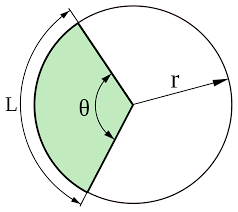The image above is a sector.

To compute the area of a sector, two essential parameters is needed and this parameters are the radius of the sector (r) and the angle of the sector (θ). You can also use the diameter of the sector (d).

The formula for calculating the area of a sector:

Area of a sector = (θ/360)[πr²]

Where;

A = Area of the Sector
r = Radius of the Sector
θ = Angle of the Sector

Let’s solve an example:
Find the area of a sector when the radius of the sector is 7 cm and the angle of the sector is 9°

This implies that;

r = Radius of the sector = 7 cm
θ = Angle of the sector = 9°

A = θ / 360 x πr2
A = (9/360)[π x 7²]
A = 0.025 x π x 49
A = 3.848

Therefore, the area of the sector is 3.848 cm2.

Calculating the Area of a Sector using Diameter and Angle of the sector.

The formula is A = θ / 360 x πd2 / 4

Where;

θ = Angle of the sector
d = Diameter of the sector

Let’s solve an example;
Find the Area of a sector when the diameter of the sector is 12 cm and the angle of the sector is 18°.

This implies that;

d = Diameter of the sector = 12 cm
θ = Angle of the sector = 18°

A = θ / 360 x πd2 / 4
A = 18 / 360 x 3.142 (144) / 4
A = 18 / 360 x 452.448 / 4
A= 18 / 360 x 113.112
A= 0.05 x 113.112
A = 5.656

Therefore, the area of the sector with diameter is 5.656 cm

How to Calculate Angle of a Sector when Area of the Sector and Radius of the Sector is Given

θ = 360 (A) / πr2

where;

r = Radius of a sector
A = Area of a sector

Let’s solve an example;
Given that the area of a sector is 15 cm2 and the radius of the sector is 5 cm. Find the angle of the sector?

This implies that;
A = Area of the sector = 15 cm2
r = Radius of the sector = 5 cm

θ = 360 (A) / πr2
θ = 360 (15) / 3.142 (5)2
θ = 5400 / 3.142 (25)
θ = 5400 / 78.55
θ = 68.746

Therefore, the angle of the sector is 68.746°.

How to Calculate Angle of a Sector when Area of the Sector and Diameter of the Sector is Given

θ = 1440 (A) / πd2

where;

d = Diameter of a sector
A = Area of a sector

Let’s solve an example;
Given that the area of a sector is 22 cm2 and the diameter of the sector is 10 cm. Find the angle of the sector?

This implies that;
A = Area of the sector = 22 cm2
d = Diameter of the sector = 10 cm

θ = 1440 (A) / πr2
θ = 1440 (22) / 3.142 (10)2
θ = 31680 / 3.142 (100)
θ = 31680 / 314.2
θ = 100.88

Therefore, the angle of the sector is 100.88°.

How to Calculate Diameter of a Sector when Area of the Sector and Angle of the Sector is Given

d = √1440 (A) / πθ

where;

θ = Angle of a sector
A = Area of a sector

Let’s solve an example;
Given that the area of a sector is 24 cm2 and the angle of the sector is 10°. Find the diameter of the sector?

This implies that;
A = Area of the sector = 24 cm2
θ = Angle of the sector = 10°

d = √1440 (A) / πθ
d = √1440 (24) / 3.142 x 10
d = √34560 / 31.42
d = √1099.936
d = 33.165

Therefore, the diameter of the sector is 33.165 cm.

How to Calculate Radius of a Sector when Area of the Sector and Angle of the Sector is Given

r = √360 (A) / πθ

where;

θ = Angle of a sector
A = Area of a sector

## How to Calculate and Solve for the Perimeter or Circumference, Radius and Diameter of a Circle | The Calculator Encyclopedia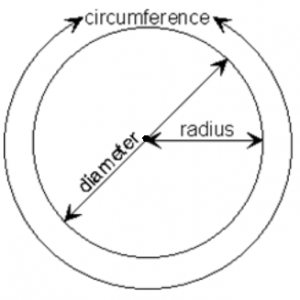The image above is a circle.

To compute the perimeter or circumference of a circle, one essential parameter is needed and this parameter is the radius of a circle (r). You can also use the diameter of a circle to compute the area of a circle (d).

The formula for calculating the perimeter or circumference of a circle is:

P = 2πr

Where:

P = Perimeter or Circumference of a circle
r = Radius of a circle

Let’s solve an example:
Find the perimeter or circumference of a circle where the radius of a circle is 8 cm.

This implies that;
r = Radius of a circle = 8 cm

P = 2πr
P = 2 x 3.142 x 8
P = 50.265

Therefore, the perimeter or circumference of a circle is 50.265 cm.

Calculating the Area of a Circle using the Diameter of a Circle.

The formula is P = πd

Where:

P = Perimeter or Circumference of a circle
d = Diameter of a circle

Let’s solve an example:
Find the perimeter or circumference of a circle where the diameter of a circle is 10 cm.

This implies that;
d = Diameter of a circle = 10 cm

P = πd
P = 3.142 x 10
P = 31.42

Therefore, the perimeter or circumference of a circle with diameter is 31.42 cm.

How to Calculate Radius of a Circle when Perimeter or Circumference of the Circle is Given

r = P /

where;

r = Radius of a circle
P = Perimeter or Circumference of a circle

Let’s solve an example:
Find the radius of a circle where the perimeter or circumference of the circle is 16 cm.

This implies that;
P = Perimeter or Circumference of the circle = 16 cm

r = P /
r = 16 / 6.284
r = 2.55

Therefore, the radius of the circle is 2.55 cm.

How to Calculate Diameter of a Circle when Perimeter or Circumference of the Circle is Given

d = P / π

where;

d = Diameter of a circle
P = Perimeter or Circumference of a circle

Let’s solve an example;
Find the diameter of a circle where the perimeter or circumference of the circle is 20 cm

This implies that;
P = Perimeter or Circumference of the circle = 20 cm

d = P / π
d = 20 / π
d = 6.365

Therefore, the diameter of the circle is 6.365 cm.

## How To Calculate and Solve for the Area, Radius and Diameter of a Circle | The Calculator Encyclopedia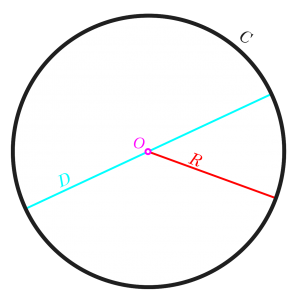The image above is a circle.

To compute the area of a circle, one essential parameter is needed and this parameter is the radius of a circle (r). You can also use the diameter of a circle to compute the area of a circle (d).

The formula for calculating the area of a circle is:

A = πr2

Where:

A = Area of a circle
r = Radius of a circle

Let’s solve an example:
Find the area of a circle where the radius of a circle is 6 cm.

This implies that;
r = Radius of a circle = 6 cm

A = πr2
A = 3.142 (6)2
A = 3.142 (36)
A = 113.10

Therefore, the area of a circle is 113.10 cm2

Calculating the Area of a Circle using the Diameter of a Circle.

The formula is A = πd2/4

Where;
A = Area of a circle
d = Diameter of a circle

Let’s solve an example:
Find the area of a circle where the diameter of the circle is 7 cm.

This implies that;
d = Diameter of a circle = 4 cm

A = πd2 / 4
A = 3.142 (4)2 / 4
A = 3.142 (16) / 4
A = 3.142 (4)
A = 12.57

Therefore, the area of a circle with diameter given is 12.57 cm2

## How to Calculate and Solve for the Centroid or Centre of Gravity of a Sphere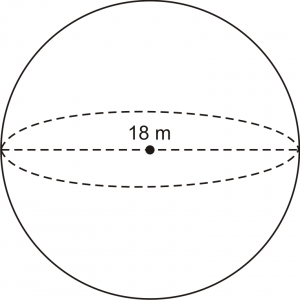The image above is a sphere and 18 m is the diameter of the sphere. In the computing of the centroid or centre of gravity of a sphere there is only one essential parameter which is the diameter of the sphere.

The formula for calculating the centroid or centre of gravity of a sphere is:

C.G. = d / 2

Where:

d is the diameter of the sphere

As always, let’s take an example:

Let’s solve an example
Find the centroid or centre of gravity of a sphere where the diameter is 18 m.

C.G. = 18 / 2
C.G. = 9

Therefore, the centroid or centre of gravity of the sphere is 9.

Nickzom Calculator – The Calculator Encyclopedia is capable of calculating the centre of gravity of a sphere at a distance from every point.

To get the answer and workings of the center of gravity or centroid of a sphere using the Nickzom Calculator – The Calculator Encyclopedia. First, you need to obtain the app.

You can get this app via any of these means: